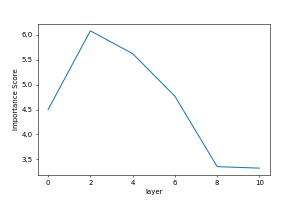This website serve as an interative tool to visualize transformer model by its latent space. The detail of the method is explained in this paper. Code is available at Here

## Introduction

Word embedding vectors can be factorized into a sparse linear combination of word factors

\begin{align*} % \vspace{-0.2in} \text{apple} =& 0.09\text{dessert}" + 0.11\text{organism}" + \\ & 0.16\text{fruit}" + 0.22\text{mobile&IT}" + 0.42\text{other}". \end{align*}

We view the latent representation of words in a transformer as contextualized word embedding. Similarly, our hypothesis is that a contextualized word embedding vector can also be factorized as a sparse linear superposition of a set of elementary elements, which we call transformer factors.

We feed many sentences into a transformer model. Then we collect the hidden state for each word from each layers. Let $X$ be set of hidden states from different layers, $\forall x \in X$, we assume $x$ is a sparse linear superposition of transformer factors: $$\label{sparse} x = \Phi \alpha + \epsilon, \ s.t. \ \alpha \succeq 0,$$

where $\Phi\in{\rm I\!R}^{d\times m}$ is a dictionary matrix with columns $\Phi_{:,c}\$, $\alpha \in{\rm I\!R}^m$ is a sparse vector of coefficients to be inferred and $\epsilon$ is a vector containing independent Gaussian noise samples, which are assumed to be small relative to $x$. Each column $\Phi_{:,c}\$ of $\Phi$ is a transformer factor and its corresponding sparse coefficient $\alpha_c$ is its activation level.

How the dictionary is learned is explained in the paper. Transformer factors $\Phi_{:,c}$ are divided into three different levels: low level, mid level and high level:

Low level transformer factors correspond to word-level disambiguation.

Mid level transformer factors correspond to sentence-level patterns.

High level transformer factors correspond to long range patterns.

The details on how to divide those transformer factors into three levels is also explained in the paper.

## Visualization

In the following box, input a number $c$ indicating the transformer factor $\Phi_{:,c}$ you want to visualize. Then click the button "" to visualize this transformer factor at a particular layer. For a transformer factor $\Phi_{:,c}$ and for a layer-$l$, the visualization is done by listing the 200 word and context with the largest sparse coefficients $\alpha^{(l)}_c$'s

### ← Enter an integer from 0 to 531, indicating the transformer factor you want to visualize.

Alternatively, you can click the three buttom below to get a random low level, mid level or high level transformer factor:

← (We generate saliency map for high level transformer factor using LIME)*This is the "importance score" for this transformer factor. The importance score reflects the average activation of this transformer factor in different layers.